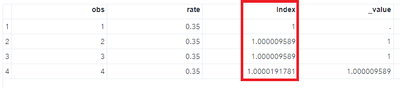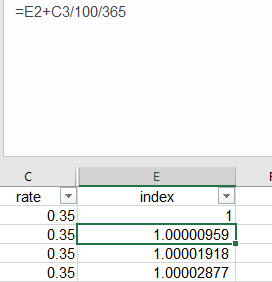## How to calculate a variable based on a lag logic

Hello,

Please find below data Have and data Want.

The index for the first observation is 1 and then it is based on the following logic

index = lag(index) + rate/100/365 ;

-------------------------------------
data Have ;
input obs rate ;
cards ;
1 0.35
2 0.35
3 0.35
4 0.35
;
run ;

----------------------------

data Want ;
input obs index ;
cards ;
1 1
2 1.00000959
3 1.00001918
4 1.00002877
;
run ;

-----------------------------

My following code test was not good, pls adivse

data next ;
set have ;
length Index 8 ;
retain index 1 ;
_value = lag(index) ;
if obs  gt 1  then index = _value + rate/100/365 ;
run ;

--------------------

This is originally from EXCEL function ( E2+C3/100/365 ) such that column E is the index and column C is the rate.

Thanks

1 ACCEPTED SOLUTION

Accepted Solutions

## Re: How to calculate a variable based on a lag logic

The problem is related to the fact that LAG is not a lookback function - it is an update FIFO queue function.   And you are updating that queue in the

`` _value = lag(index) ;``

statement prior to the recalculation of INDEX in the

``if obs  gt 1  then index = _value + rate/100/365 ;``

statement.  So the recalculated INDEX value does not get into the queue in a timely way.

But in this case, you don't need LAG at all, since you are retaining INDEX.

``````data next ;
set have ;
length Index 8 ;
retain index 1 ;
if obs gt 1 then index = index + rate/100/365;
run ;
``````

--------------------------
The hash OUTPUT method will overwrite a SAS data set, but not append. That can be costly. Consider voting for Add a HASH object method which would append a hash object to an existing SAS data set

Would enabling PROC SORT to simultaneously output multiple datasets be useful? Then vote for
Allow PROC SORT to output multiple datasets

--------------------------
5 REPLIES 5

## Re: How to calculate a variable based on a lag logic

Hi,

Perhaps I have not understood what you're asking, but when I ran your code, the index column that you calculated appears to match your want data:Were you looking for something else?

Thanks & kind regards,

Amir.

## Re: How to calculate a variable based on a lag logic

Hi,

Each observation should get a different value of index.

However, in the test code both observation 2 and 3 get the same value of index.

This is wrong.

Thanks

## Re: How to calculate a variable based on a lag logicA little clarification:

The calculation above in Excel is correct.

How can we do it in SAS ?

## Re: How to calculate a variable based on a lag logic

The problem is related to the fact that LAG is not a lookback function - it is an update FIFO queue function.   And you are updating that queue in the

`` _value = lag(index) ;``

statement prior to the recalculation of INDEX in the

``if obs  gt 1  then index = _value + rate/100/365 ;``

statement.  So the recalculated INDEX value does not get into the queue in a timely way.

But in this case, you don't need LAG at all, since you are retaining INDEX.

``````data next ;
set have ;
length Index 8 ;
retain index 1 ;
if obs gt 1 then index = index + rate/100/365;
run ;
``````

--------------------------
The hash OUTPUT method will overwrite a SAS data set, but not append. That can be costly. Consider voting for Add a HASH object method which would append a hash object to an existing SAS data set

Would enabling PROC SORT to simultaneously output multiple datasets be useful? Then vote for
Allow PROC SORT to output multiple datasets

--------------------------

## Re: How to calculate a variable based on a lag logic

Many Thanks !

Discussion stats
• 5 replies
• 198 views
• 0 likes
• 3 in conversation